Function Repository Resource:

# NFourierSinCoefficient

Find a numerical approximation for a Fourier sine coefficient of a function

Contributed by: Wolfram Research
 ResourceFunction["NFourierSinCoefficient"][expr,t,n] gives a numerical approximation to the nth coefficient in the Fourier sine series expansion of expr.

## Details and Options

The numerical approximation to the nth coefficient in the Fourier sine series expansion of expr is by default defined to be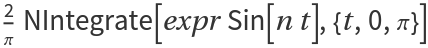, where n must be an integer.
Different choices for the definition of the Fourier sine series expansion can be specified using the option FourierParameters.
With the setting FourierParameters{a,b}, the nth coefficient computed by ResourceFunction["NFourierSinCoefficient"] is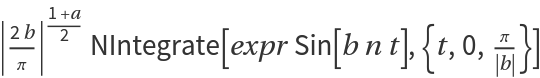.
The parameter b in the setting FourierParameters{a,b} must be numeric.
In addition to the option FourierParameters, ResourceFunction["NFourierSinCoefficient"] can also accept the options available to NIntegrate. These options are passed directly to NIntegrate.

## Examples

### Basic Examples (3)

Calculate the numerical approximation to a Fourier sine coefficient:

 In:=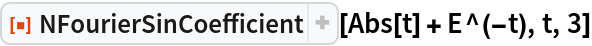Out=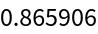Compare with the answer from symbolic evaluation:

 In:=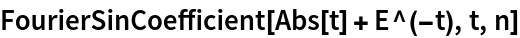Out=In:=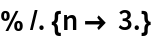Out=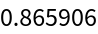Repeat the computation, using a different definition of the Fourier sine transform:

 In:=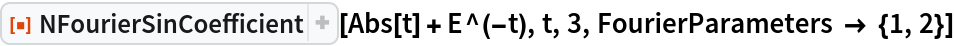Out=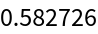## Requirements

Wolfram Language 11.3 (March 2018) or above

## Version History

• 1.0.0 – 26 April 2019